Previous Chapter

### Markets--Putting It All Together

PART 1 - EQUILIBRIUM PRICE AND QUANTITY

In this chapter, we will combine what we learned about demand and supply curves in Chapters 3, 4, and 5 with the idea of trade discussed in Chapter 6, in order to understand how prices and quantities are determined.

In Chapter 3, we saw how the behavior of an individual consumer led to a demand curve, a relation between the price at which he could buy a good and the quantity he chose to buy. In most markets, all customers pay about the same price, so we can talk of a single market price and a single demand curve, representing the total demand of all consumers for the good as a function of its price. Since total quantity demanded at any price is the amount I want to buy at that price plus the amount you want to buy at that price plus the amount he wants to buy at that price plus . . . , the market demand curve is the horizontal sum of the individual demand curves, as shown in Figure 7-1.

In Chapter 4, I showed how we could analyze consumption in terms of continuously variable goods by using rate of consumption instead of number of units consumed--cookies per week rather than cookies. When we are considering the combined demand of a large number of people, we have a second reason for treating goods and curves as continuous. For lumpy goods such as automobiles, for which individual demand curves are step functions rather than curves (you buy one automobile or two, not 1.32 automobiles), even a very small drop in price will make a few consumers (out of millions) decide to buy a car instead of not buying one.

In Chapter 5, I showed how individual supply curves could be derived, starting with a producer's output rates for different goods plus either his marginal disvalue for labor curve or the indifference curves showing his preferences with regard to leisure and income. Just as the market demand curve is the horizontal sum of individual demand curves, so the market supply curve is the horizontal sum of the individual supply curves; having seen how to derive the individual supply curves for a good, we also know how to derive its total supply curve.Market demand curve. The market demand curve is the horizontal sum of individual demand curves, since total quantity demanded at any price is the sum of my quantity demanded at that price plus your quantity demanded at that price plus . . . .

We are now ready to put supply curves and demand curves together. Figure 7-2a shows supply and demand curves for widgets, a hypothetical commodity consumed mostly by the authors of economics textbooks. The particular curves shown in the figure happen to be straight; as you may have guessed by now, the term curve, in the language of economists and mathematicians, includes straight lines. The vertical axis of the diagram is price, the horizontal axis is quantity; any point on the diagram, such as A, represents a quantity and a price (QA and PA). What will the market price be and what quantity will be produced and consumed at that price?

As any experienced guesser could predict, the answer is point E, where the supply and demand curves cross. The interesting question is why.

Suppose the price were P1 on Figure 7-2a. At that price, producers wish to produce and sell a quantity Q1, while consumers only wish to purchase a (smaller) quantity Q1'. Some of the producers find themselves with widgetsthat they cannot sell. In order to get rid of them, the producers are willing to cut the price. Price falls. It continues to do so as long as the quantity supplied is greater than the quantity demanded.

Suppose, instead, that the price were P2 on Figure 7-2a. Now producers wish to produce a quantity Q2, while consumers wish to purchase a (larger) quantity Q2'. Consumers cannot consume goods that are not produced, so some of them are unable to buy what they want. They are willing to offer a higher price, so they bid the price up. Figure 7-2b shows what is happening in terms of the marginal value curve of one such consumer. At P2 he would like to buy q2' but finds he can only buy q2. At that quantity, his marginal value for another widget is P' > P2; he is willing to pay any price up to P' in order to get another widget, so the price is bid up.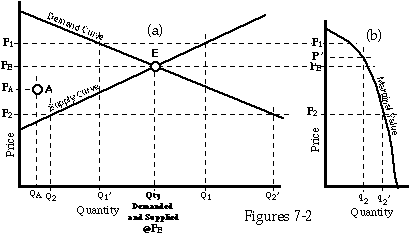Market equilibrium. At point E, price = PE; quantity demanded equals quantity supplied. At lower prices, less is supplied; individuals are consuming quantities for which MV > P, as shown on Figure 7-2b, and so are willing to offer a higher price for additional quantities.

If the price is below PE, the price for which quantity supplied and quantity demanded are equal, it will be driven up; if it is above PE, it will be driven down. So PE is the equilibrium price. But PE is the price for which quantity supplied (at price PE) equals quantity demanded (at price PE), so it is the price at the point where the two curves cross.

The idea of an equilibrium--a situation in which a system generates no forces that tend to change it--is common to many different sciences. It is often useful to distinguish three different sorts of equilibria. A stable equilibrium is one in which, if something does move the system slightly away from the equilibrium, forces are set in motion that move it back again. An unstable equilibrium is one in which, if something moves the system slightly away from the equilibrium, forces are set in motion that move it even further away. A metastable equilibrium is one in which, if something moves the system slightly away from the equilibrium, no forces are set in motion at all--it remains in the new position, which is also an equilibrium.

The three sorts of equilibria can be illustrated with a pencil. Hold the pencil by the point, with the eraser hanging down. It is now in a stable equilibrium--if someone nudges the eraser end to one side, it swings back. Balance the pencil on its point on your finger. It is now in an unstable equilibrium--if someone nudges it, it will fall over. Lay the pencil (a round one) down on the table. It is now in a metastable equilibrium--nudge it and it rolls over part way and remains in its new position. One sometimes encounters people, either human or feline, who appear to be in metastable equilibrium.

The equilibrium illustrated in Figure 7-2a and again in Figure 7-3a is stable--if you move the price and quantity away from E, forces are set in motion that move them back. In zone I (on Figure 7-3a), quantity demanded is greater than quantity supplied, so price goes up; in zone III, quantity demanded is less than quantity supplied, so price goes down. In zone II, the quantity being produced is more than producers want to produce at that price, so they reduce their output; in zone IV, for similar reasons, they increase their output. The restoring forces are shown by the arrows in Figure 7-3a.

Figure 7-3b is a similar but less plausible diagram in which the supply curve is falling rather than rising. The result is an unstable equilibrium. If price is above P*, quantity demanded is larger than quantity supplied, which drives the price up even further; similarly, if price is below P*, quantity demanded is less than quantity supplied, driving the price down. Figure 7-3c is an (implausible) diagram showing a range of metastable equilibria, with quantity equal to Q* and price between P1 and P2.

We now have a simple rule for combining a supply curve and a demand curve to get a market price and quantity. The equilibrium occurs at the intersection of the two curves and is stable if the demand curve is falling and the supply curve rising--as we shall always assume that they are, for reasons discussed in Chapters 3, 4, and 5. We shall now use this rule to analyze the effects of shifts in demand and supply curves.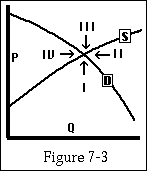Stable equilibrium. The arrows show the directions of the forces moving the system back to equilibrium.

#### Elasticity--A Brief Digression

The effect on price and quantity of shifts in supply and demand curves depends, among other things, on how steep the curves are. Economists find it useful to discuss the steepness of curves in terms of their elasticity. The price elasticity of a demand or supply curve, at a point, is defined as the percentage change in quantity divided by the percentage change in price. If, for instance, a 1 percent increase in price results in a 1 percent increase in quantity supplied, we have:

Percent change in quantity/percent change in price = 1%/1% = 1.

So, in this case, the supply elasticity is 1. Similarly, if we graphed quantity demanded against income with price held fixed (a rising curve for a normal good, a falling curve for an inferior good), we would define the income elasticity of the curve at a point as the percentage change in quantity divided by the percentage change in income that caused it.

For the purposes of this chapter, all you need to know is that very elastic means that a small change in price results in a large change in quantity while very inelastic means that a large change in price results in only a small change in quantity. The limiting cases are perfectly elastic (a horizontal supply or demand curve) and perfectly inelastic (a vertical curve). We will discuss the idea of elasticity in more detail in Chapter 10.

#### Shifting Curves

Figure 7-4a shows a supply-demand diagram with a shift in the demand curve from D1 to D2. One can imagine this resulting from a change in tastes (widgets become more popular), weather (it has been a hot summer and widgets work better in hot weather), the price of other goods (widgets use a lot of widget oil, the price of which has just fallen), consumer incomes, expected future prices, or whatever. The demand curve has shifted out. Demand has increased--at every price, the new quantity demanded is larger than the old. The result is to move the equilibrium point from E1 to E2. Both equilibrium price and equilibrium quantity increase.

In Figure 7-4b, the shift occurs in the supply curve. Supply has increased, perhaps because labor has become more expensive or raw materials cheaper; at every price, the new quantity supplied is larger than the old. The result is an increase in quantity but a decrease in price. These results are completely general; as long as demand curves slope down and supply curves up, an increase in demand will increase both price and quantity, while an increase in supply will increase quantity but decrease price. Decreases in demand or supply have the opposite effects. You should be able to convince yourself of these relationships by examining Figures 7-4a and 7-4b.

It is important, in order to avoid confusion, to distinguish between changes in supply (the supply curve shifting, as in Figure 7-4b) and changes in quantity supplied. In Figure 7-4a, the supply curve stays fixed but the quantity supplied increases from Q1 to Q2. A supply curve (or a demand curve) is a relation between price and quantity; if the price changes because of a shift in the demand curve, the new price results in a new quantity supplied, even if the supply curve does not change. In Figure 7-4c, on the other hand, both the supply curve and the demand curve shift, while the quantity supplied stays exactly the same. The shift in the supply curve in this example is just enough to cancel the effect of the change in price, so the new price on the new supply curve yields the same quantity supplied as the old price on the old supply curve. One could easily enough construct other examples where a shift in both curves changed quantity but not price, or changed both.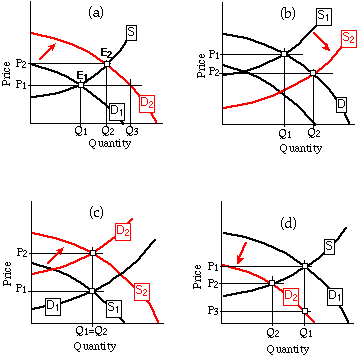Figures 7-4

The effects of shifts in supply and demand curves. In Figures 7-4a and 7-4d, the demand curve shifts. In Figure 7-4b, the supply curve shifts. In each case, quantity demanded, quantity supplied, and price all change as a result. In Figure 7-4c, both the demand curve and the supply curve shift; price changes, but quantity stays the same.

One should distinguish similarly between changes in demand and changes in quantity demanded. By being careful about both distinctions, one can avoid some of the worst absurdities of newspaper discussions of economics. Consider, for example, the following paradoxes:

"If demand increases, that bids up the price, so increased demand is associated with increased price. But if price rises, that decreases demand, so decreased demand is associated with increased price."

If demand increases, that bids up the price, but the increased price drives demand back down again."

If demand decreases, that drives down the price, which drives down the supply, which brings the price back up."

Most such confusions can be avoided by drawing the relevant curves and distinguishing carefully between shifts of the curves (demand or supply moving out or in) and movements along the curves (quantity demanded or supplied changing because of a change in price). If the demand curve shifts, as in Figure 7-4a, while the supply curve stays fixed, that is a change in demand, which changes price, which changes quantity supplied--but supply (the supply curve) is still the same. A change in supply, as in Figure 7-4b, changes price and quantity demanded but not demand.

The first two paradoxes are illustrated on Figure 7-4a. An increase in demand (the demand curve shifts out) raises price; the increased price reduces quantity demanded below what it would have been if the demand curve had shifted but the price had remained the same (Q3). The resulting quantity demanded (Q2), although less than Q3, is more than the old quantity demanded (Q1). Q2 must be greater than Q1 because quantity demanded is equal to quantity supplied, the supply curve has not shifted, and a higher price applied to the same supply curve must result in a larger quantity supplied.

The third paradox is illustrated on Figure 7-4d. A decrease in demand (the demand curve shifts in) lowers the price; quantity supplied is now lower than it would have been at the old price (Q2 < Q1). But quantity supplied is equal to quantity demanded (at the new price on the new demand curve), so there is no reason for the price to go back up. What is true is that if the lower price had not reduced the quantity supplied, price would have had to fall even further (to P3 on Figure 7-4d) in order for quantity supplied and quantity demanded to be equal. It is only in this sense that the "reduced supply" (actually reduced quantity supplied) "brings the price back up" (reduces the amount by which the price falls).

Figures 7-5a through 7-5f show some interesting extreme cases. In Figures 7-5a and 7-5b, the supply curves are perfectly elastic. On Figure 7-5a, the industry will produce any quantity at a price P (or any higher price, but the price can never get higher since as soon as it does quantity supplied exceeds quantity demanded) and no quantity at any lower price. A shift in demand (Figure 7-5a) has no effect on price; it simply results in a different quantity at the old price. A shift in supply from S1 to S2, both perfectly elastic (Figure 7-5b), changes the price by the (vertical) amount of the shift and the quantity by the effect of that price change on quantity demanded along the old demand curve.

In Figures 7-5c and 7-5d, demand is perfectly elastic. Consumers are willing to buy an unlimited quantity at a price P on Figure 7-5c (or any lower price, but . . .) and nothing at any higher price. A shift in supply (Figure 7-5c) leaves price unaffected but changes quantity by the (horizontal) amount of the shift. A shift in demand (Figure 7-5d) changes price by the vertical amount of the shift and quantity by the effect of the price change on the quantity supplied.

In Figure 7-5e, the supply curve is perfectly inelastic--the quantity supplied does not depend on price. The supply of land is often thought of as perfectly inelastic--there are a certain number of square miles on the earth's surface, and that is that. The supply of labor is also perfectly inelastic--if we include the part of your labor that you supply to yourself (leisure). Defined in this way, the supply of labor is 24 hours per day times the population. It is fixed, at least over the short term. What we normally call the supply of labor is this minus the demand by the owners of the labor. I do not work 24 hours per day because I choose to consume some of my labor myself. Both of these examples will come up again in Chapter 15.

With a perfectly inelastic supply curve, a shift in the demand curve results in a corresponding change in price and no change in quantity, as shown in Figure 7-5e. With a perfectly inelastic demand curve (a "need" in the sense discussed and rejected in Chapter 2), a shift in the supply curve has a similar effect, as shown in Figure 7-5f.

One of the differences between economics as done by economists and economics as done by journalists, politicians making speeches, and others, is that the non-economists often speak as though all supply and demand curves were perfectly inelastic. This is the same disagreement that I discussed earlier in the context of "needs" vs "wants." The non-economist tends to think of the demand for water as "the amount of water we need" and assumes that the alternative to having that amount of water is people going thirsty. But, as you should know from answering one of the questions in Chapter 4, only a tiny fraction of the water we consume is drunk. While the demand for drinking water may be highly inelastic over a wide range of prices, demand for other uses is not. If the price of water doubles, it pays farmers to use water more sparingly for irrigation, chemical firms to use less of it in their manufacturing processes, and homeowners to fix leaky faucets more promptly than before. Nobody dies of thirst, but total consumption of water falls substantially.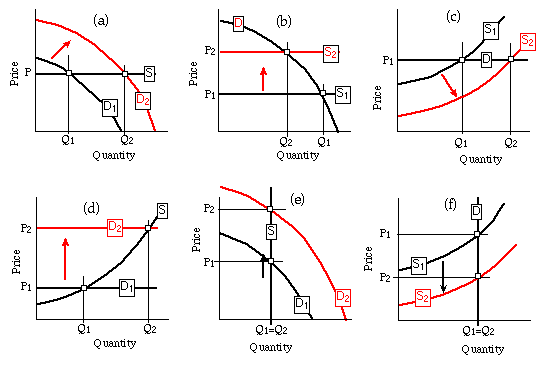Figures 7-5

The effect of shifts when one curve is either perfectly elastic or perfectly inelastic. When one curve is perfectly elastic, a shift in the other changes quantity but not price (Figures 7-5a and 7-5c); when one curve is perfectly inelastic, a shift in the other changes price but not quantity (Figures 7-5e and 7-5f).

#### Who Pays Taxes?

We are now ready to start answering one of the questions frequently asked of economists; the number of weeks and pages it has taken us to get this far may explain why answers that fit in a newspaper column or a 30-second news report are generally unsatisfactory. The question is "Who really pays taxes?" When the government imposes a tax on some good, does the money come out of the profits of those who produce the good or do the producers pass it along to the consumers in higher prices?

Suppose, for example, that the government imposes a lump sum sales tax of \$1/widget; for every widget that is sold, the producer (assumed to be the seller--there are no middlemen at this stage of the analysis) must pay the government \$1. The result is to shift the supply curve up by \$1, from S1 to S2, as shown in Figure 7-6.

Why does a sales tax shift the supply curve in this way? What matters to the producer is how much he gets, not how much the consumer pays. A price of \$6/widget with the tax gives the producer the same amount for each widget sold as a price of \$5/widget without the tax. So he will produce the same quantity of widgets at \$6/widget after the tax is imposed as he would have produced at \$5 before, and similarly for all other prices. Each quantity on the new supply curve corresponds to a price \$1 higher than on the old, so the supply curve is shifted up by \$1.

This does not mean that the market price goes up \$1. If it did, producers would produce the same amount as before the tax; consumers, at the higher price, would consume less than before, so quantity supplied would be greater than quantity demanded. If, on the other hand, price did not rise at all, quantity demanded would be the same as before the tax. Quantity supplied would be less (since producers would be getting a dollar less per widget), so quantity supplied would be less than quantity demanded. As you can see on Figure 7-6, the price rises, but by less than a dollar. All of the tax is "paid" by the producer in the literal sense that the producer hands the government the money, but in fact the price paid by the consumer has gone up by a and the price received by the producer has gone down by b.

To see why b on the figure equals the decrease in the price received by the producer, note that if the market price had gone all the way up to P3 = P1 + \$1, the producer's receipts, after paying the \$1 tax, would still be P1 per widget, just as before the tax was imposed. Since the price only goes to P2 = P1 + a, the producer's receipts per widget (after tax) have fallen by the difference between P3 and P2, which is b. Put algebraically, we have:The effect of a \$1 tax on widgets collected from the producers. The supply curve shifts up from S1 to S2 due to the tax; equilibrium price rises by a, from \$5/widget to \$5.60/widget. Quantity falls from 1.1 million widgets per year to 1 million widgets per year.

P3 - P2 = (P1 + \$1) - (P1 + a) = \$1 - a = b.

As you can see by examining Figure 7-6, the way in which the burden of the tax is divided between consumers and producers depends on the slopes of the supply and demand curves. Figures 7-7a and 7-7b show two extreme cases. In Figure 7-7a, the supply curve S is perfectly inelastic; you cannot see the shift in S, since shifting a vertical line up moves it onto itself. Since quantity and the demand curve stay the same, the price must stay the same, so the entire burden of the tax is borne by the producers; it is sometimes asserted that this is true of a tax on land. In Figure 7-7b, the demand curve is perfectly inelastic, and the price increases by the full dollar; the entire burden of the tax is borne by the consumers. Most real-world cases fall between these two extremes.

In Figure 7-8a, the initial demand and supply curves for widgets are the same as in Figure 7-6; what has changed is the form of the tax. Instead of taxing producers, the government has decided to tax consumers. For every widget you buy, you must pay the government \$1. The result is to shift the demand curve instead of the supply curve--from D1 down to D2. The number of widgets you choose to buy depends on what it costs you to buy them--the price plus the tax. Suppose that before the tax was imposed you chose to buy 12 widgets at \$5 apiece. With the tax, the price at which you would choose to buy 12 is \$4, since at that price you are again paying \$5/widget--\$4 to the producer and \$1 to the government.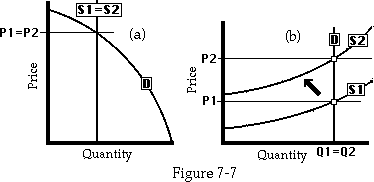The effect of a tax when demand or supply is perfectly inelastic. In Figure 7-7a, the supply curve is perfectly inelastic and the entire burden of the tax is borne by the producers. In Figure 7-7b, the demand curve is perfectly inelastic and the entire burden is borne by the consumers.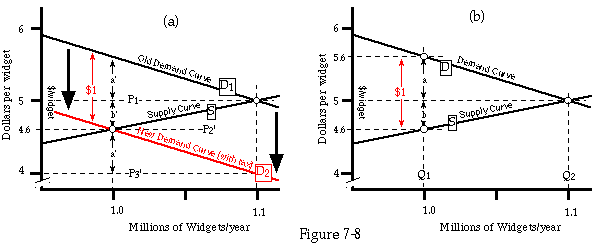Two ways of graphing the effect of a \$1 tax on widgets. In Figure 7-8a, the tax is collected from consumers, shifting the demand curve down from D1 to D2. In Figure 7-8b, the demand curve is a function of price paid (market price plus any tax on consumers), and the supply curve is a function of price received (market price minus any tax on producers). The figure could show a \$1 tax on either producer or consumer.

This is an application of the principle that costs are opportunity costs, discussed in Chapter 3. The cost to you of buying a widget for \$5 is the loss of the goods you would otherwise have bought with the money. So widgets at \$5 with no tax cost you the same amount as widgets at \$4 with a \$1 tax, payable by the consumer; in each case you give up, for each widget purchased, the opportunity to buy \$5 worth of something else. Since the cost to you of each widget is the same in both cases and since you decide how many widgets to purchase on the basis of cost and value, as described in the derivation of the individual demand curve in Chapter 4, you buy the same quantity in both cases. So does everyone else. So the total quantity demanded is the same at a price of \$4 with the tax as it would be without the tax at a price of \$5, and similarly for all other prices. The demand curve shifts down by \$1--the amount of the tax.

Looking at Figure 7-8a, you can see that as a result of the shift in the demand curve, the price received by the producer has gone down by an amount b' and the amount paid per widget by the consumers has gone up by a'. To see why a' is the increase in what consumers are paying per widget, note that if price had fallen by a full dollar, to P3', the consumers would have been no worse off--what they paid on the tax they would have made up on the lower price, making the cost to them of each widget the same as before. In fact, price has only fallen to P2' > P3'; hence the increase in what consumers are paying for each widget is P2' - P3' = (P1' - b') - (P1' - \$1) = \$1 - b' = a' .

If you compare Figure 7-8a with Figure 7-6, you can see that they are essentially the same figure; b = b' and a = a'. Figure 7-8a is simply Figure 7-6 with everything shifted down by \$1. The reason is that in Figure 7-6, the price shown on the vertical axis is price after tax, since the tax is paid by the producer; in Figure 7-8a, it is price before tax, since the tax is paid by the consumer. The difference between price before tax and price after tax is the amount of the tax: \$1. In both cases, quantity supplied is determined by price received by the producer, quantity demanded by price paid by the consumer, and the effect of the tax is to make price paid by the consumer \$1 higher than price received by the producer.

A third way of describing the same situation is shown in Figure 7-8b. Here supply is shown as a function of price received, demand as a function of price paid. Before the tax was instituted, market equilibrium occurred at a quantity (Q1) for which price received was equal to price paid; after the tax was instituted, market equilibrium occurs at a quantity (Q2) for which price received is a dollar less than price paid, with the difference going to the government.

If you look carefully at Figures 7-6, 7-8a, and 7-8b, you should be able to see that they are all the same; the only thing that changes from one to another is what is shown on the vertical axis. The figures are the same not just because I happen to have drawn them that way but because they have to be drawn that way; all three describe the same situation. The cost of widgets to the consumers (which is what matters to them), the amount received by the producers per widget sold (which is what matters to them), and the quantity of widgets sold are all the same whether the tax is "paid" by producers or consumers. How the burden of the tax is really distributed is entirely unaffected by who actually hands over the money to the government!

#### And for the Real Cost of Taxes . . .

The previous section started with the question of who really pays taxes. It seems we now have the answer. Using a supply-demand diagram, we can show how much of the tax is passed along to the consumer in the form of higher prices and how much appears as a reduction in the (after-tax) receipts of the producer. In any particular case, the answer depends on the relative elasticity of the supply and demand curves--on how rapidly quantity demanded and quantity supplied change with price, as indicated by the slope of the curves S and D on our diagrams.

We have answered a question, but it is not quite the right question. We know by how much the tax raises the cost of widgets to the consumer and by how much it lowers the revenue received by the producer for each widget he sells, but that is not quite the same thing as how much worse off it makes them. The cost to the consumer is not merely a matter of how much money he spends; it also depends on what he gets for it.

To see this in a particularly striking way, imagine that the government decides to impose a tax of \$1,000/widget. Production and consumption of widgets drop to zero. The government receives nothing; producers and consumers pay nothing. Does that mean that a tax of \$1,000/widget costs consumers (and producers) nothing?

Obviously not. The consumers could have chosen to consume zero widgets before the tax increase--the fact that they actually consumed 1,100,000 widgets at a price of \$5/widget (Figure 7-9) indicates that they preferred that number of widgets at that price to zero widgets; hence the tax has made them worse off. Our mistake was in assuming that the cost of the tax to the consumers was simply the number of widgets they bought times the increase in the price of the widgets. We should also have included the loss due to the reduced consumption of widgets.

Let us now consider a more reasonable tax--\$1/widget instead of \$1,000/widget. This makes the cost of widgets to consumers \$5.60 and the quantity demanded and supplied 1,000,000, as shown by P2 and Q2 on Figure 7-9. Before there was any tax at all, consumers bought 1,100,000 widgets per year; after the \$1 tax was imposed, their consumption went down to 1,000,000. The extra 100,000 widgets were worth at least \$5 apiece (which is why consumers bought them before the tax was imposed, when the price of widgets was \$5 each) but less than \$5.60 (which is why they no longer buy them when the price goes up to \$5.60). The consumers are worse off by the benefit they no longer get from purchasing those 100,000 widgets per year at \$5 apiece, as well as by the increased price they must pay for the 1,000,000 widgets per year they continue to purchase. Similarly, the producers are worse off by the profits they would have made on the additional 100,000 widgets, as well as by lost revenue on the 1,000,000 widgets they still produce.

What we left out of our initial analysis of the cost of taxation was consumer (and producer) surplus, which was introduced in Chapter 4 (and 5) to measure the benefit to a consumer (producer) of being able to purchase (sell) as much as he wanted of a good at a particular price. Before the tax, the consumer could purchase as many widgets as he wanted at \$5 apiece; afterwards he could purchase as many as he wished at \$5.60 apiece. The cost to him of the tax is the difference between the consumer surplus he received in the first case and the consumer surplus he received in the second. This is shown in Figure 7-9. The entire shaded and colored area of Figure 7-9 is the consumer surplus received before the tax. The darkly shaded area is the consumer surplus received after the tax. The colored area is the difference between the two--the cost of the tax. It is divided into two regions. R1 is a rectangle whose height is the increase in the price and whose width is the quantity of widgets being consumed after the tax (1,000,000 per year). R2 is an (approximate) triangle representing the lost consumer surplus on the 100,000 widgets per year that were consumed before the tax and are not consumed after the tax. R1 is the amount of the tax paid by the consumers, in the sense discussed earlier in this chapter--the price increase times the quantity being consumed after the tax is imposed. R2 is part of the excess burden of the tax. R1 is a loss to the consumers and an equal gain (in tax revenue) for the government; R2 is a loss for the consumers with no corresponding gain for anyone. It has often been argued that the ideal system of taxes is that which minimizes excess burden, thus collecting a given amount of revenue at the lowest possible cost.The effect on consumer surplus of a \$1 tax on widgets. The entire shaded and colored area is consumer surplus before the tax; the dark shaded area is consumer surplus after the tax. The colored area is the cost the tax imposes on consumers. R1 is revenue collected by the tax; R2 is excess burden.

Figures 7-10a and 7-10b show that the relation between R1 and R2 depends on the shape of the demand curve. If it is very flat (demand is highly elastic), then the increased price due to the effect of the tax (from P1 to P2) results in a large reduction in quantity demanded and a large loss of consumer surplus relative to the amount of tax revenue collected. P3 on the diagram is the price at which quantity demanded is zero (the choke price); for a tax that raises the price of the good that high, R2 is substantial (the entire consumer surplus at P1) and R1 is zero. The effect of such a tax is all excess burden and no revenue at all.

If, on the other hand, the slope of the demand curve is very steep (demand is highly inelastic) , as shown in Figure 7-10b, then the increased price results in only a small decrease in consumption, and R2 is small compared to R1. In the limiting case of perfectly inelastic demand, there is no reduction in consumption and hence no excess burden.

This has sometimes been used as an argument for taxing "necessities" instead of "luxuries." The idea is that demand for necessities is very inelastic (you "have to have them") while demand for luxuries is very elastic; hence taxes on necessities produce little excess burden compared to taxes on luxuries. Attempts to actually measure price elasticity of demand for different goods do not always bear out this presumption; the demand for cigarettes, for example, which are usually thought of as luxuries (and sinful ones at that--hence the object of "sin taxes"), seems to be relatively inelastic. In any case, even if taxes on necessities do minimize excess burden, there remains the objection that taxes on necessities "hurt the poor" while taxes on luxuries "soak the rich"--and the latter is generally more popular, at least as a political slogan, than the former.

So far in this discussion, I have concentrated on the cost of the tax to the consumer. A similar analysis could be applied to the producer, with producer surplus substituted for consumer surplus. The result would be similar; for consumers as well as for producers, the cost of the tax includes an element of excess burden, and the relation between excess burden and the rest of the cost to the producers depends on the elasticity of the supply curves.The effect of elasticity of the demand curve on the relation between revenue and excess burden. A very elastic demand curve (Figure 7-10a) produces a high ratio of excess burden to revenue; a very inelastic demand curve (Figure 7-10b) produces a low ratio.

In discussing the excess burden imposed by taxes, or anything else that depends on elasticity of supply and demand, it is important to distinguish between short-run and long-run effects. Elasticities of both supply and demand are usually greater in the long run than in the short. If the price of gasoline rises, the immediate response of the consumer is to drive less. Given a longer time to adjust, he can also arrange a car pool, buy a smaller car, or move closer to his job. If the price of heating oil rises, he can adjust, in the short run, only by turning down his thermostat. In the long run, he can improve the insulation of his house or move to a warmer climate.

In the short run, the producer is stuck with his existing factory. If price falls, he may still prefer producing at the lower price to scrapping his machinery. In the longer term, supply is more elastic; at the lower price, it will no longer be worth maintaining the machines or buying new ones as the old ones wear out. If price rises, his short-run response is limited to trying to squeeze more output from the existing factory. In the longer run, he can build a bigger factory.

For all of these reasons, elasticities are generally greater in the longer run. High elasticity implies high excess burden, so the excess burden of a tax is likely to become larger, relative to the amount collected, as time goes on. A famous example is the window tax in London some centuries ago, which led to a style of houses with few windows. A similar and more recent example was a tax on houses in New Orleans that depended on the number of stories at the front of the house. That is supposed to be the origin of the "camelback" houses--one story in front, two in back. In the long run, dark houses in London and higher building costs in New Orleans were part of the excess burden of those taxes.

#### Landlords and Tenants--An Application of Price Theory

Suppose the government of Santa Monica decides that since landlords are bad and tenants good, every landlord must pay each of his tenants \$10/month. In the short run, this benefits the tenants and hurts the landlords, since rents are set by contracts that usually run for a year or so; as long as the tenant is paying the same rent as before and receiving an additional \$10, he is better off than before. In the longer run, however, the supply and demand curves for apartments are shifted by the new requirement, changing the equilibrium rent. The effect is shown in Figure 7-11a.

From the standpoint of the landlord, the new requirement is simply a tax of \$10/month on each apartment rented. What matters to the landlord in deciding whether to rent out an apartment (as opposed to occupying it himself, turning it into a condominium, letting the building fall apart, not building it in the first place, or whatever other alternatives he has) is how much he ends up getting, not how much the tenant initially pays him. Since he has to give \$10 back to the tenant, he actually gets rent minus \$10. So the supply curve is shifted up by \$10; at a rent of \$510 per apartment per month, the quantity of apartments offered to rent is the same as it would have been before at a rent of \$500/month.

From the standpoint of the tenant, the \$10 is a subsidy--a negative tax. A positive tax would shift the demand curve down by the amount of the tax; the effect of the negative tax is to shift the demand curve up by \$10. Whatever quantity of housing each tenant would have chosen to rent before at a price of \$500/month (instead of buying a house, sharing an apartment with a friend, or moving to Chicago), that is now the quantity he will choose to rent if the rent is \$510, since \$510 in rent minus \$10 from the landlord is a net cost to him of \$500. If the rent is less than \$510, he will choose to rent more housing than he rented before at \$500; if the rent is more than \$510, he will rent less.Effect of regulations on the rental market. Figure 7-11a shows the effect of a compulsory \$10 transfer from landlords to tenants. Figure 7-11b shows the effect of requiring landlords to provide tenants with six months' notice. The requirement is equivalent to a \$10 tax on landlords and a \$5 subsidy to tenants.

Figure 7-11a shows the result; for simplicity we are treating housing as if it were a simple continuous commodity like water, and defining price and quantity in terms of some standard-sized apartment. Since both supply and demand curves shift up by \$10, their intersection shifts up by \$10 as well. The new equilibrium rent is precisely \$10 higher than the old, so the law neither benefits the tenant nor hurts the landlord.

If this result seems paradoxical to you, you are a victim of what I earlier called naive price theory. Once the assumption that prices are handed down from heaven in some mysterious manner is replaced by an understanding of how they are determined, the result is not only possible but obvious. If every time you pay the rent, the landlord is required to go through a ceremony of extracting one \$10 bill and giving it back to you, there is no reason why that requirement should affect the rent you really pay and he receives.

Let us now consider a different law--one that seems at first less arbitrary. The city government decides that it is unfair for landlords to "force" tenants to sign lease contracts that are "biased" in favor of the landlords, so it passes a law requiring landlords to give tenants six months' notice before evicting them, even if the tenants have agreed in the lease to some shorter period. Again we consider the effect after enough time has passed so that rents have had a chance to adjust themselves to the new equilibrium, as determined by the supply and demand curves after the change.

Suppose the landlords are all identical. The requirement of six months' notice increases their operating costs, since it makes it harder to evict undesirable tenants. From their standpoint, it is equivalent to a tax. Suppose it is equivalent to a tax of \$10. Suppose, in other words, that landlords are indifferent between having to provide each tenant with six months' notice and having to pay a \$10/month tax on each apartment. The supply curve for apartments shifts up by \$10, as shown in Figure 7-11b.

From the standpoint of the tenants (who we will also assume are all identical to each other), the additional security of the six months' requirement is worth something; an apartment with that security is worth more than one without it. It is thus equivalent to them to receiving a subsidy--a negative tax. Suppose that it is equivalent to a subsidy of \$5/month. Just as in the previous case, the result is to shift the demand curve up; the same tenant who was willing to pay \$500/month for an apartment without six months' tenure is willing to pay \$505 for one with the additional security. The curve shifts up by \$5, as shown in Figure 7-11b. The result is similar to the \$10 transfer shown on Figure 7-11a, but the demand curve shifts up only \$5 instead of \$10.

Looking at the figure, you can see that the new price is higher than the old by more than \$5 and less than \$10. This is not an accident. The exact price depends on the slope of the curves, but (as you should, with a little effort, be able to prove) the increase must be more than the smaller shift and less than the larger. Since the law increases the costs to landlords by more than it increases rents, landlords are worse off. Since it increases the value of the apartment to tenants by less than it increases rents, tenants are also worse off!

In setting up the problem, I assumed that the six months' notice requirement cost the landlords more than it was worth to the tenants. What happens if we make the opposite assumption? Suppose the law imposes a cost of \$5 (shifting the supply curve up by \$5) and a benefit of \$10 (shifting the demand curve up by \$10). In that case, as you should be able to demonstrate, the increase in rent is again between \$5 and \$10. Both parties are better off as a result of the law--the landlord gets an increase in rent greater than the increase in his costs, while the tenant pays an increase in rent less than the value to him of the improved contract.

In this case, however, the law is unnecessary. If there is no law setting the terms for rental contracts, a landlord who is renting out his apartment (without six months' security) for \$500/month will find it in his interest to offer his tenant the alternative of the same apartment with six months' security at, say, \$509/month. The tenant will accept the offer, since (by our assumption) he prefers \$509 with security to \$500 without; the landlord will be better off, since it only costs him, on average, \$5/month to provide the security. Hence, even without the law, all rental contracts will provide for six months' notice before eviction. So in this case, the law has no effect; it forces the landlords to do something they would do anyway.

More generally, it will pay the landlord to include in the lease contract any terms that are worth more to the tenant than they cost him--and adjust the rent accordingly. Given that he has done so, any requirement that he provide additional security (or other terms in the contract) forces the landlord to add terms to the lease that cost him more than they are worth to the tenant. The ultimate result is a rent increase that leaves both landlord and tenant worse off than before.

In proving this result, I made a number of simplifying assumptions. One was that the cost per apartment imposed by such a requirement on the landlord did not depend on how many apartments he was renting out; the requirement, like a lump sum tax, shifted the supply curve up by the same amount all along its length. I also made the equivalent assumption for the tenants--that security was worth the same amount per apartment independent of how much apartment was consumed (the horizontal axis of the diagrams should really represent not number of apartments but amount of housing rented--rooms, square feet of apartment space, or some similar measure). Dropping these assumptions would make the diagrams and the analysis substantially more complicated. With some effort, one could construct situations where the requirement shifts the supply and demand curves in a way that benefits tenants at the expense of landlords, or landlords at the expense of tenants, but there is no particular reason to expect either to happen.

A second assumption was that all landlords were identical to each other, and similarly for all tenants. Dropping these assumptions changes the results somewhat. To see why, imagine that you are a landlord who is unusually good at recognizing good tenants. Offering six months' security costs you nothing--you never rent an apartment to anyone you will ever want to evict. Assume that the situation, with regard to the tenants and the other landlords, is as shown in Figure 7-11b. If there is no legal restriction on contracts, you find that by offering security you can get a rent of \$505/month instead of \$500; since the security costs you nothing, you do so. After the law changes to force all landlords to offer security, you find that the market rent, for apartments with the (required) six months' security, is more than \$505 (as shown in Figure 7-11b). The restriction has actually helped you, by forcing your competitors (the other landlords) to add a feature (security) to their product that was expensive for them to produce but inexpensive for you. Their higher costs decreased the market supply curve and increased the market price, benefiting you.

One could construct similar cases involving tenants. The interesting point to note is that the effect of legal restrictions on contracts between landlords and tenants is not, as one might at first expect, a redistribution from one group (landlords) to another (tenants). Insofar as the groups are uniform, the restrictions either have no effect or injure everyone; insofar as the members of the groups differ from each other, the restriction may also result in redistribution within the groups--benefiting some members of one or both of the groups at the expense of other members of the same group.

One of the difficulties in teaching (and learning) economics is that so much of it seems to be simply plausible talk about familiar subjects. That is an illusion. In the course of this chapter, I have given proofs of two very implausible results--that it does not matter whether a tax is collected from producers or consumers and that restrictions on rental contracts "in favor" of tenants actually hurt both tenants and landlords.

The fact that economics seems to be merely plausible talk about familiar things poses a serious danger to the student. He may go through the first half of the course nodding his head from time to time at what seem like reasonable statements by the text or the professor, only to discover at the midterm that he has somehow failed to learn most of the structure of ideas that the lectures and text were supposed to be teaching--even though it all seemed to make sense when he heard it.

The best way to find out for yourself whether you really understand the ideas is to try to apply them to numerical examples. That is the significance of such examples. Whether you do or do not become an economist, it is unlikely that you will ever be faced with any real-world equivalent of a numerical problem on an economics exam; the real world rarely provides us with accurate graphs of supply and demand curves. What you will be faced with--whether as an economist or a participant in the economy--are problems similar to the problem of figuring out whether a restriction on lease contracts benefits or harms tenants. To do so, you must learn economics, not merely learn about economics; numerical problems are a way to check whether or not you have done so. In exactly the same sense, the right way to find out whether you have learned typing is not to see if you can tell someone about it, or remember what you have been taught, but to try to type something--even if it is only "The quick brown fox jumped over the lazy dog." Many of the problems in an economics text are about as closely related to the ways in which you will actually use economics as the quick brown fox is to the things you eventually expect to type--and serve much the same function.

#### PART 2 - SUPPLY AND DEMAND--SOME GENERAL POINTS

In the first part of this chapter, we put together what we had done in Chapters 3-6 in order to show how equilibrium price and quantity are determined by demand and supply curves. We then applied the analysis to a variety of real-world issues. In the second part of the chapter, we will look back at what we have just done in order to clarify some points and avoid some common misunderstandings.

#### Mechanism versus Equilibrium

In economics (and elsewhere), there are often two different ways of approaching a problem--to work through a (possibly infinite) series of changes or to look at what the situation must be when all changes have ceased. The latter is often much easier than the former.

Consider a simple supply-demand problem. At a price of \$1, purchasers of eggs wish to buy 1,000 eggs per week and producers wish to produce 900. The first way of analyzing the problem might go as follows:

Step 1: There are only 900 eggs to be purchased. The consumers bid against each other until they have driven the price up to \$1.25; at that price, they only want to buy 900 eggs.

Step 2: At the new price, producers want to produce 980 eggs per week. They do so. They find that at \$1.25, they cannot sell that many, so they compete against each other (by cutting the price) until the price falls to \$1.05; at that price, consumers will buy 980 eggs.

Step 3: At \$1.05, producers only want to produce 910 eggs per week. They do so. Consumers bid against each other . . .

As you can see, there are several things wrong with this approach. To begin with, the series may go on forever, with the prices gradually converging; indeed, with some demand and supply curves, the series would diverge--the swings in price would get wider and wider. Furthermore, the analysis assumes that producers and consumers will always base their decisions on what the price just was instead of trying to estimate what it is going to be. Once you drop that assumption, there are many possible sequences of events, depending on the detailed assumption you make about how producers predict the future. The alternative approach goes as follows:

If quantity supplied is greater than quantity demanded, the price will fall; if less, the price will rise. Price will therefore tend toward the point at which the two are equal. This is the equilibrium price--the intersection of supply and demand.

#### Shortages, Surpluses, and How to Make Them

To most non-economists, a shortage is a fact of nature--there just isn't enough. To an economist, it has almost nothing to do with nature. Diamonds are in very short supply--yet there is no diamond shortage. Water is very plentiful; the average American consumes, directly or indirectly, more than 1000 gallons per day. Yet we see water shortages.

The mistake is in assuming that "enough" is a fact of nature--that we need a particular amount of land, water, diamonds, oil, or whatever. In fact, the amount we choose to consume (and that producers choose to produce) depends on the price; what we think of as our "need" is usually our quantity demanded at the price we are used to paying. A shortage occurs not when the amount available is small but when it is less than the amount we want; since the latter depends on price, a shortage simply means that a price is too low--below the level where quantity supplied would equal quantity demanded. Frequently this is the result of either government price control (gas and oil prices in the early seventies, for example) or the refusal by government to charge the market price for something it supplies (urban water). Sometimes it is the result of producers who misestimate demand (particular models of cars) and are unwilling or unable to adjust price or output quickly. An obvious example is where the seller is bound by an advertised price and finds that at that price he runs out.

One interesting case of a relatively stable supply-demand disequilibrium (a surplus rather than a shortage) occurred many years ago in Hong Kong. Rickshaws are small carts drawn by one person and used to transport another--a sort of human-powered taxi. They used to be common in Hong Kong. Casual observation suggested that the drivers spent most of their time sitting by the curb waiting for customers--quantity supplied was much larger than quantity demanded. Why?

The explanation appears to be that many of the customers were tourists from countries where the wage level was, at that time, much higher than in Hong Kong. The price it seemed natural to them to offer to pay was far above the price at which supply would have equaled demand. People were attracted into the rickshaw business until the daily income (one fourth of the day working for a high hourly payment, three fourths of the day sitting around) was comparable to that of other Hong Kong jobs. It is worth noticing that the tourists who paid \$4HK for a ride that represented \$1HK worth of labor were worse off by \$3HK than if they had paid the lower price but that there was no corresponding gain to the recipients.

#### The Invisible Demand Curve

A careless reading of an economics textbook may give the impression that economists are people who go around measuring supply and demand curves and calculating prices and quantities from them. This is a complete misunderstanding. What we observe are prices and quantities. To the extent that we know anything about particular demand curves, it is mostly deduced from such observations. For the most part, supply curves and demand curves are used not as summaries of information (like a table of atomic weights) but as analytical tools, ways of understanding the mechanism by which prices are determined.

Indeed, demand and supply curves are in a sense unobservable. We observe that this year there was a certain amount of wheat grown and it sold at a certain price. We make similar observations for other years. Can we plot the corresponding points on a graph and call them a demand curve ("When price was \$1, demand was 4,000,000 bushels; when price was . . .")? No. If the demand curve and supply curve had stayed the same from year to year, price and quantity would have stayed the same as well. Since they did not, at least one of the curves must have shifted, and perhaps both. If the demand curve stayed the same and the supply curve shifted around (changes in the weather affecting the supply of wheat, for instance), then the observed points trace out the demand curve (Figure 7-12a), but if it was demand that shifted and supply that stayed fixed, then we have a graph of the supply curve instead (Figure 7-12b). If both curves shifted, we have a graph of neither (Figure 7-12c).

#### Demand or Supply?

One of the original puzzles of economics was whether price was determined by the value of a good to the purchaser (demand) or the cost of production (supply). You are now in a position to see that the answer is both. If the supply curve is horizontal at a price P, then P is the market price, whatever the demand curve may be--unless the quantity demanded at that price is zero, in which case nothing is sold and there is no market price. If demand is horizontal at a price P, then that will be the market price--whatever the supply curve (with the same exception). In the normal case, where neither line is horizontal, a shift in either will change the price.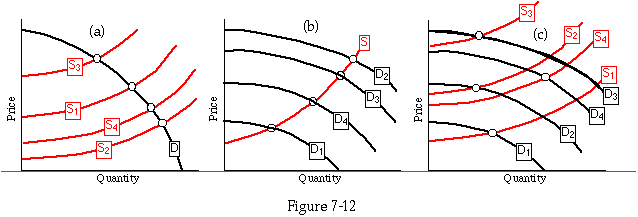Invisible curves. What we observe is only the intersection of a supply and a demand curve. If the supply curve is shifting (Figure 7-12a), the intersections map out the demand curve; if the demand curve is shifting (Figure 7-12b), they map out the supply curve; and if both are shifting (Figure 7-12c), they show neither.

The statement "Price is determined by both value to the consumer and cost of production" is also true in a more complicated sense; it is in a sense true that "Price is equal to both value to the consumer and cost of production"--even in the extreme case of a horizontal supply curve, where price is determined entirely by supply.

How can this be true? It is true if value and cost mean marginal value and marginal cost. The consumer, faced with the opportunity to consume as much as he wants at a price P, chooses to consume that quantity of the good at which his marginal value for an additional unit is just equal to P. We saw that in Chapter 4, where we derived the demand curve from the consumer's marginal value curve. So price equals value--not because value determines price but because price (at which the good is available) determines quantity (that the consumer chooses to consume) and quantity consumed determines (marginal) value. Seen from the other side, the producer, able to sell all he wants at a price P, expands output until his marginal cost of production (the marginal disvalue per hour of labor to him divided by the number of units he produces in an hour) is P. We saw that in Chapter 5 and will see it again, in the case of a firm rather than an individual producer, in Chapter 9. So price equals cost--not because cost determines price but because price (at which he can sell the good) determines quantity (that he produces) which determines (marginal) cost.

In considering a single consumer or a single producer, we may take price as given, since his consumption or production is unlikely to be large enough to influence it significantly. Considering the entire industry (made up of many producers) and the entire demand curve (made up of many individual demand curves), this is no longer true. The market price is that price at which quantity demanded equals quantity supplied. At the quantity demanded and supplied at that price, price equals marginal cost equals marginal value. Demand and supply curves jointly determine price and quantity; quantity (plus demand and supply curves) determines marginal value and marginal cost.

#### Warning

There are two somewhat subtle mistakes that I have found students often make in interpreting the material of this chapter. The first is a verbal mistake with regard to demand. It is easy to think of "my demand increasing" as meaning "I want it more." But demand (the demand curve) is a graph of quantity demanded as a function of price. If your demand increases, that means that at any price, you want more of it--not that you want it more. How much you want it is your marginal value, not your demand; it depends, among other things, on how much of it you have. That the two curves (demand and marginal value) are the same is not a matter of definition (they mean quite different things) but something that we proved in Chapter 4.

The other mistake has to do with why supply curves shift. We are used to thinking of prices as the result of bargaining between buyer and seller, with each claiming that the price he wants is fair. It is tempting to imagine that when a tax is imposed, the reason price rises is that the seller tells the buyer, "Look here. My costs have gone up, so it is only fair for you to let me raise my price." The buyer replies, "I agree that it is fair for you to raise your price, but I should not have to bear the whole cost of the tax, so let us compromise on a price increase that transfers part of the tax to me and leaves you paying the rest."

Such an imaginary dialogue has nothing to do with the process I have been describing in this chapter, and almost nothing to do with how prices are really determined. To begin with, I am assuming a market with many buyers and many sellers; each individual, in deciding how much to buy or sell, takes the price as given, knowing that nothing he does can much affect it. In such a context, bargaining has no place. If you do not like my price, I will sell to someone who does; if nobody will buy at the price I am asking, I must have made a mistake about what the market price was.

When a tax is imposed on the producers, each producer revises his calculation of how much it is in his interest to produce. Before the tax, when the price was, say, \$10, he produced up to the point where producing an additional widget cost him as much, in time and effort, as \$10 was worth to him. When a \$1 tax is imposed, he finds that each additional widget is bringing him only \$9--\$10 for the widget minus \$1 for the tax. He is in the same situation as if there were a \$9 price and no tax, so he reduces his production to what he would have produced if the price were \$9 and there were no tax. He is not trying to bargain with anyone--he is simply maximizing his welfare under changed circumstances. All other producers act similarly--some by reducing production, some by leaving the industry and producing something else instead. Quantity supplied (at \$10) is now less than quantity demanded, so price rises. As it rises, producers increase the amount they find it in their interest to produce; consumers decrease the amount they find it in their interest to consume. The price continues to rise until it reaches a level at which the quantity producers wish to produce is the same as the quantity consumers wish to consume.

#### PROBLEMS

1. Figures 7-13a and 7-13b each show demand curves for two individuals; in each case, draw the combined demand curve.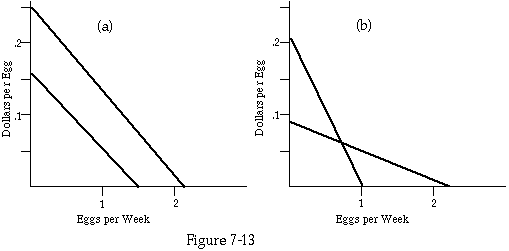Demand curves for Problem 1.

2. Figure 7-14 shows the supply curve for avocados. A tax of \$0.50/avocado is imposed. Draw the new supply curve.

3. Figure 7-15 shows the demand curve for peanut butter. A consumption tax is imposed; every purchaser must pay the government \$0.40 for every jar of peanut butter purchased. Draw the new demand curve for peanut butter.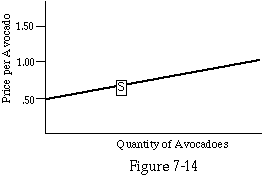Supply curve for Problem 2.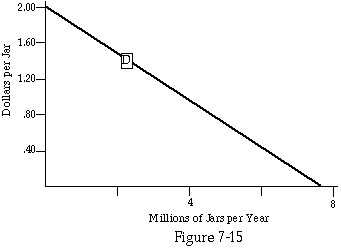Demand curve for Problem 3.

4. Figure 7-16 shows demand and supply for potatoes in 1925. For each quote below, redraw the figure showing the change described. The parts are alternatives, not successive events. The authors of the quotes may be assumed to know as much economics as the average newspaper reporter. You may explain your answers if you wish.

a. In 1926, the invention of the french fry caused a great increase in the demand for potatoes. The result was to increase the price of potatoes. The increased price increased the supply, which drove the price back down again.

b. In 1926, bad weather decreased the potato supply, driving up the price. The higher price decreased the demand, which drove the price down.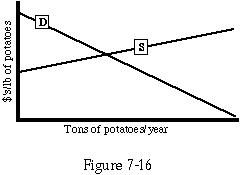Supply and demand curves for Problem 4.

5. Figure 7-17 shows the supply and demand curves for bananas.

a. What are the equilibrium price, quantity, and total consumer expenditure?

For parts (b-g), answer the following questions:

i. What is the equilibrium price? Quantity? Consumer expenditure for bananas?

ii. How much better or worse off are consumers than in part (a) (no tax)? Producers?

iii. How much revenue does the government get?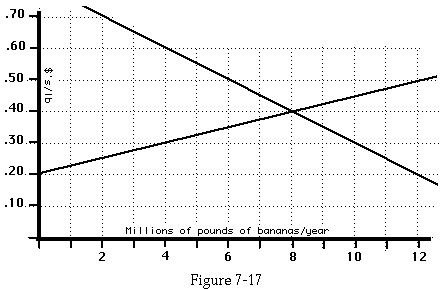Supply and demand curves for Problem 5.

b. Consumers must pay a tax of \$0.10/pound on bananas.

c. Producers must pay a tax of \$0.10/pound on bananas.

d. Producers pay and consumers receive a tax of \$0.10/pound.

e. Producers and consumers each pay a tax of \$0.05/pound.

f. The government requires all bananas to be labeled with the date they were picked. This results in an increased cost to producers of \$0.08/pound. Consumers are indifferent between unlabeled bananas at a price P and labeled bananas at a price P plus \$0.03.

g. As in (f), except that the cost to the producers is \$0.03 and the value to the consumers \$0.08. Comment on your results.

6. Can a tax lower the total expenditure of consumers on bananas and at the same time hurt the consumers? If so, give an example. Explain.

(Note: Each of parts (b-h) is independent; in each case, we start with the same initial situation, make one change, and evaluate the result.)

7. Work out the effect of a tax in the case of a perfectly elastic supply curve. In the case of a perfectly elastic demand curve.

8. What are the relations between price elasticity of demand and supply and excess burden to the producers? Demonstrate your results with figures similar to Figures 7-5a through 7-5f, 7-10a, and 7-10b.

9. What is the exact mathematical relation between P2 and P3 in Figure 7-6 and P2' and P3' in Figure 7-8a?

10. I asserted that one could, with some effort, construct a situation where a restriction on rental contracts actually benefited landlords at the expense of tenants. Do so. You should assume that for every quantity, the demand curve shifts up less than the supply curve (the restriction costs the landlord more than it benefits the tenant), but you need not assume that the shift is uniform along the curve.

11. Social Security taxes are paid half by the employer and half by the worker. What do you think is the significance of that division? How would things be different if the tax were entirely on the worker or entirely on the employer or divided in some other way between them? Prove your answer.

12. Why do you think the present division of Social Security payments between employer and employee exists?

13. In discussing the surplus of rickshaws, I claimed that the high price paid by customers to rickshaw drivers was a cost to the customer but not a gain to the driver. Explain.

Next Chapter